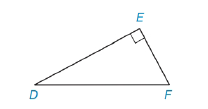Chapter 5.4, Problem 9EElementary Geometry For College St...

7th Edition
Alexander + 2 others
ISBN: 9781337614085

Solutions

Chapter
SectionElementary Geometry For College St...

7th Edition
Alexander + 2 others
ISBN: 9781337614085
Textbook Problem

Find EF if:a) D F = 17 and D E = 15 .b) D F = 12 and D E = 8 2 .Exercises 7-10

To determine

(a)

To Find:

The length of EF¯.

Explanation

Given DF=17 and DE= 15,

And figure,

From the figure,

ΔDEF is a right triangle with right angle E

From the Pythagorean Theorem,

The square of the length of hypotenuse of a right triangle is equal to the sum of the square of the lengths of the other two sides.

From the figure, DF¯ is the hypotenuse,

DF2=EF2+D

To determine

(b)

To Find:

The length of EF¯.

Still sussing out bartleby?

Check out a sample textbook solution.

See a sample solution

The Solution to Your Study Problems

Bartleby provides explanations to thousands of textbook problems written by our experts, many with advanced degrees!

Get Started

Sketch the graphs of the equations in Exercises 512. xy=2x3+1

Finite Mathematics and Applied Calculus (MindTap Course List)

Elementary Technical Mathematics

2. Define the terms population, sample, parameter and statistic.

Statistics for The Behavioral Sciences (MindTap Course List)

81/2

Applied Calculus for the Managerial, Life, and Social Sciences: A Brief Approach

Evaluate the limit, if it exists. limt01+t1tt

Single Variable Calculus: Early Transcendentals

The absolute maximum value of f(x) = 6x − x2 on [1, 7] is: 3 9 76 −7

Study Guide for Stewart's Single Variable Calculus: Early Transcendentals, 8th

Prove the idempotent law for disjunction, ppp.

Finite Mathematics for the Managerial, Life, and Social Sciences